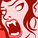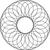Announcements
Product Release Webinar: Qlik Insider airing December 6! REGISTER TODAY!
cancel
Showing results for
Did you mean:Contributor II

## Apply a filter to a variable

I created this variable in the load script:

set vSaleCur = sum( {<#(vFlag) = {'1'}, Actual_only = {'Yes'}, Comparative=, Year = {#(=#(vMaxYear))}>} SALES);

let vSaleCur = replace('\$(vSaleCur)', '#', chr(36));

Now I'm using it in a chart as en expression, like this:

=\$(vSalesCur)

Now I need to apply a new filter when using the variable as an expression in the chart so it disregards the filter by month, something like this:

=sum( {<Month=>} \$(vSalesCur))

I do not want to add the filter when creating the variable and I do not want to create a new variable with the new filter included because this is one example that I need to do with a lot of other variables

So...

NOT THIS:

set vSaleCur = sum( {<#(vFlag) = {'1'}, Actual_only = {'Yes'}, Comparative=, Month=, Year = {#(=#(vMaxYear))}>} SALES);

NOT THIS EITHER:

set vSaleCurNew = sum( {<#(vFlag) = {'1'}, Actual_only = {'Yes'}, Comparative=, Month=, Year = {#(=#(vMaxYear))}>} SALES);

Any thoughts?

Labels (3)

• ### Variable definition

2 RepliesCreator III

Something like this?

Script

set vSaleCur = sum({<#(vFlag) = {'1'}, Actual_only = {'Yes'}, Comparative =, Year = {#(=#(vMaxYear))} #(=replace('#1', '|', ','))>} SALES);

let vSaleCur = replace('\$(vSaleCur)', '#', chr(36));

Front End

Adding the set modifier that ignores the selections made on the Month field...

\$(vSaleCur(| Month =))

\$(vSaleCur( ))Contributor II
Author

Hi JGMD,

I want to avoid changing the script.  I want to know if there is a way to change the variable in the front end keeping the back-end untouched.  So the question is if there is a way to apply further filtering in a variable already calculated?

If I'm using \$(vSalesCur), how can I wrap it around a new expression that allows me to add a new filter to it?

Something like =(<\$(vSalesCur), Month=>)Community Browser